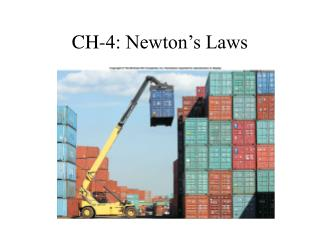# CH-4: Newton’s Laws - PowerPoint PPT PresentationDownload PresentationCH-4: Newton’s Laws

CH-4: Newton’s LawsDownload Presentation## CH-4: Newton’s Laws

- - - - - - - - - - - - - - - - - - - - - - - - - - - E N D - - - - - - - - - - - - - - - - - - - - - - - - - - -
##### Presentation Transcript

1. CH-4: Newton’s Laws

2. Moving a chair

3. Brief History • Aristotle (384-322 B.C) • Galileo Galilei (1564-1642) • Isaac Newton (1642-1727)

4. Aristotle (384-322 B.C) • Believed that a force had to act for an object to move. • Did not distinguish acceleration from velocity. • Believed that a heavy object would fall more quickly than a lighter object. • Earth-centered model of the solar system.

5. What force makes this baseball move?

6. Galileo Galilei (1564-1642) • Advocated Copernicus’s Sun-centered model of the solar system. • Showed that heavy and light objects fell at the same rate. • Argued that no force is required to maintain motion. • Developed mathematical description of motion.

7. Isaac Newton (1642-1727) • Laws of motion, can be used to analyze motion of ordinary objects. • Not valid for speeds close to the speed of light. Need to use the theory of relativity. • Not valid for atomic sized particles. Need to use quantum mechanics.

8. Newton’s First Law of Motion

9. Newton’s First Law of Motion An object continues in a state of rest or in a state of motion at a constant speed along a straight line, unless compelled to change that state by a net force.

10. The Tablecloth Trick

11. Q11 • Will a bullet fired in outer space slow down? (no gravity nor air resistance)

12. Newton's Second Law of Motion When a net external force F acts on an object of mass m, the acceleration a that results is directly proportional to the net force and has a magnitude that is inversely proportional to the mass. The direction of the acceleration is the same as the direction of the net force.

13. Newton's Second Law of Motion • Equation Form

14. Same force applied to a bowling ball and a tennis ball

15. E8

16. 4.3 Mass and Weight The weight of an object on the earth is the gravitationalforce that the earth exerts on the object. The weight always acts downward, toward the center of the earth. On another astronomical body, the weight is the gravitational force exerted on the object by that body. SI Unit of Weight: : newton (N) Weight = Mass x Gravity

17. 4.4 Newton’s Third Law

18. 4.4 Newton’s Third Law • If object A exerts a force on object B, object B exerts a force on object A that is equal in magnitude but opposite in direction to the force exerted on B.

19. Identifying Forces • Textbook resting on a table

20. Identifying Forces

21. The road pushes the car

22. What forces are involved in moving a chair?

23. What forces are involved in moving a chair?

24. Riding an Elevator

25. Does a sky diver continue to accelerate?

26. Does a sky diver continue to accelerate?

27. Motion of Connected Objects

28. try this box 4.3

29. Newton’s Third Law Illustrated